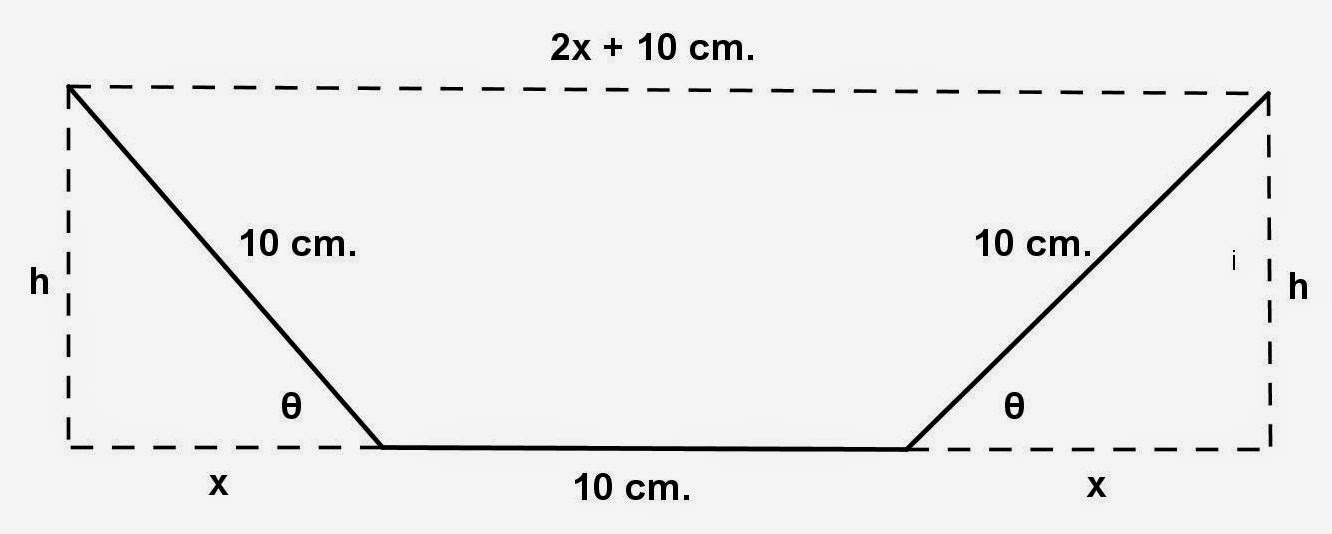Showing posts with label Differential Calculus. Show all posts
Showing posts with label Differential Calculus. Show all posts

## Monday, October 13, 2014

### Tangent Lines and Normal Lines, 5

Category: Differential Calculus

"Published in Vacaville, California, USA"

Show that the curvehas no tangent line with slope 4.

Solution:

Consider the given curve

As we know that the slope of any curve is equal to the first derivative of the equation of any curve with respect to the independent variable which is x in most cases. Take the derivative of the given equation with respect to x, we have

If the slope of a curve is dy/dx = 4 which is also the slope of a tangent line, then the above equation becomes

From the resulting equation, the values of x will be the x values of the intersection of a curve and a tangent line. By using quadratic formula, the values of x are

Since the values of x are imaginary numbers or complex numbers, then there's no tangent line for the given curve. The given curve did not intersect with the tangent line of a given slope.

## Sunday, October 12, 2014

### Maximum and Minimum Problems, 9

Category: Differential Calculus

"Published in Vacaville, California, USA"

A rain gutter is to be constructed from a metal sheet of width 30 cm. by bending up one-third of the sheet on each side through an angle θ. How should θ be chosen so that the gutter will carry the maximum amount of water?Photo by Math Principles in Everyday Life

Solution:

From the given figure, it is a cross section of a gutter in a form of isosceles trapezoid because the opposite sides as well as the opposite angles of the lower base are congruent.

In the given word problem, maximum amount means maximum volume. Since the given figure is a cross section and the height of a gutter is not given, then it is a maximum area.

If the length of the opposite sides of a trapezoid as well as the opposite angles at the lower base are given, then we can solve for the altitude and the length of the upper base.Photo by Math Principles in Everyday Life

Since the two opposite triangles at the opposite sides of a trapezoid are right triangles, then we can use the trigonometric functions as follows

The area of a cross section of a gutter which is an isosceles trapezoid is

Take the derivative on both sides of the equation with respect to θ, we have

Since we want to get the maximum amount of water or maximum area of a cross section of a gutter, then set dA/dθ, we have

but

then the above equation becomes

Equate each factors to zero and solve for the value of θ.

Since the value of θ is a straight angle, then we cannot accept this as an answer. Let's consider the other factor and solve for the value of θ, we have

Since the value of θ is an acute angle, then we can consider this one as an answer.